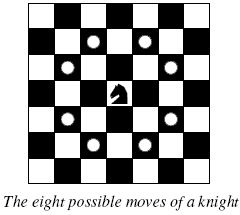# 2253. A Knight's Journey

The knight is getting bored of seeing the same black and white squares again and again and has decided to make a journey around the world. Whenever a knight moves, it is two squares in one direction and one square perpendicular to this. The world of a knight is the chessboard he is living on. Our knight lives on a chessboard that has a smaller area than a regular $8 \times 8$ board, but it is still rectangular. Can you help this adventurous knight to make travel plans?Find a path such that the knight visits every square once. The knight can start and end on any square of the board.

### 输入格式

The input begins with a positive integer $n$ in the first line. The following lines contain $n$ test cases. Each test case consists of a single line with two positive integers $p$ and $q$, such that $1 \leq p \times q \leq 26$. This represents a $p \times q$ chessboard, where $p$ describes how many different square numbers $1, \ldots, p$ exist, $q$ describes how many different square letters exist. These are the first $q$ letters of the Latin alphabet: $A, \ldots$

### 输出格式

The output for every scenario begins with a line containing Scenario #i:, where i is the number of the scenario starting at $1$. Then print a single line containing the lexicographically first path that visits all squares of the chessboard with knight moves followed by an empty line. The path should be given on a single line by concatenating the names of the visited squares. Each square name consists of a capital letter followed by a number.

If no such path exist, you should output impossible on a single line.

### 样例

Input
3
1 1
2 3
4 3

Output
Scenario #1:
A1

Scenario #2:
impossible

Scenario #3:
A1B3C1A2B4C2A3B1C3A4B2C4


136 人解决，189 人已尝试。

250 份提交通过，共有 795 份提交。

3.4 EMB 奖励。